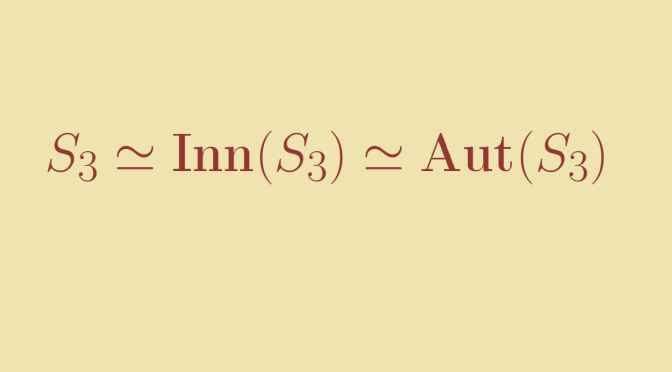# A group isomorphic to its automorphism group

We consider a group $$G$$ and we look at its automorphism group $$\text{Aut}(G)$$. Can $$G$$ be isomorphic to
$$\text{Aut}(G)$$?
The answer is positive and we’ll prove that it is the case for the symmetric group $$S_3$$.

Consider the morphism $\begin{array}{l|rcl} \Phi : & S_3 & \longrightarrow & \text{Aut}(S_3) \\ & a & \longmapsto & \varphi_a \end{array}$
where $$\varphi_a$$ is the inner automorphism $$\varphi_a : x \mapsto a^{-1}xa$$. It is easy to verify that $$\Phi$$ is indeed a group morphism. The kernel of $$\Phi$$ is the center of $$S_3$$ which is having the identity for only element. Hence $$\Phi$$ is one-to-one and $$S_3 \simeq \Phi(S_3)$$. Therefore it is sufficient to prove that $$\Phi$$ is onto. As $$|S_3|=6$$, we’ll be finished if we prove that $$|\text{Aut}(S_3)|=6$$.

Generally, for $$G_1,G_2$$ groups and $$f : G_1 \to G_2$$ a one-to-one group morphism, the image of an element $$x$$ of order $$k$$ is an element $$f(x)$$ having the same order $$k$$. So for $$\varphi \in \text{Aut}(S_3)$$ the image of a transposition is a transposition. As the transpositions $$\{(1 \ 2), (1 \ 3), (2 \ 3)\}$$ generate $$(S_3)$$, $$\varphi$$ is completely defined by $$\{\varphi((1 \ 2)), \varphi((1 \ 3)), \varphi((2 \ 3))\}$$. We have 3 choices to define the image of $$(1 \ 2)$$ under $$\varphi$$ and then 2 choices for the image of $$(1 \ 3)$$ under $$\varphi$$. The image of $$(2 \ 3)$$ under $$\varphi$$ is the remaining transposition.

Finally, we have proven that $$|\text{Aut}(S_3)|=6$$ as desired and $$S_3 \simeq \text{Aut}(S_3)$$.